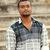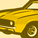# QlikView App Dev

Discussion Board for collaboration related to QlikView App Development.

Announcements
See why Qlik was recognized for the seventh year in a row – and discover how we can help you tackle your data integration challenges. Get the report
cancel
Showing results for
Did you mean:Contributor III

## Dynamically adding columns to the Straight table.

I have 50 columns in a table. I need any 10 columns to be displayed as default because having entire 50 columns would make the appearance messy , as it will lead to a lot of scrolling towards right in the straight table. So any 10 columns as default initially  and options like expanding or list box to add other 40 columns to the table when required.

My efforts so far

I created a inline table of the same original table and placed this inline table in a list box with only 40 Fields.

then I created a straight table with 9 Dimensions and 1 Date measure which are not there in list box. Now I am stuck what should i write the enable condition for Dimension.

or is there any all together a different work around for this.

1 Solution

Accepted SolutionsCreator III

Concat (PARTICIPANT_DIMENSION, '|')  and Concat (PARTICIPANT_MEASURE, '|')

Those functions create string with dimensions and measures.

For example if you have column

PARTICIPANT_DIMENSION

FIRST_NAME,

SECOND_NAME,

STREET

Concat (PARTICIPANT_DIMENSION, '|') will create the following string FIRST_NAME|SECOND_NAME|STREET|

SubStringCount function returns number of substrings in the string, so you in case of

SubStringCount (Concat (PARTICIPANT_DIMENSION, '|'), 'FIRST_NAME') it will return 1.

When you select FIRST_NAME in PARTICIPANT_DIMENSION substringcount returns 1 - "1" is true, so you will see your FIRST_NAME as dimension.

The same logic works with expressions, but I would recommend you to use alphabet letters instead of numbers if you have more than ten measures.

SubStringCount (Concat (PARTICIPANT_MEASURE, '|'), A)

Because for measures you will have following string 1|2|3|4|5|6|7|8|9|10| and if you select first measure in your measure list, you will see the 1 and 10 measure.

Thanks,

Vitalii

11 RepliesCreator III

Hi Harish,

Thanks,

VitaliiContributor III
Author

I have gone through that article @Vitalii Chuprina, Could you please explain these two conditions.

=SubStringCount (Concat (PARTICIPANT_DIMENSION, '|'), 'FIRST_NAME')

=SubStringCount (Concat (PARTICIPANT_MEASURE, '|'), 1)Creator III

Concat (PARTICIPANT_DIMENSION, '|')  and Concat (PARTICIPANT_MEASURE, '|')

Those functions create string with dimensions and measures.

For example if you have column

PARTICIPANT_DIMENSION

FIRST_NAME,

SECOND_NAME,

STREET

Concat (PARTICIPANT_DIMENSION, '|') will create the following string FIRST_NAME|SECOND_NAME|STREET|

SubStringCount function returns number of substrings in the string, so you in case of

SubStringCount (Concat (PARTICIPANT_DIMENSION, '|'), 'FIRST_NAME') it will return 1.

When you select FIRST_NAME in PARTICIPANT_DIMENSION substringcount returns 1 - "1" is true, so you will see your FIRST_NAME as dimension.

The same logic works with expressions, but I would recommend you to use alphabet letters instead of numbers if you have more than ten measures.

SubStringCount (Concat (PARTICIPANT_MEASURE, '|'), A)

Because for measures you will have following string 1|2|3|4|5|6|7|8|9|10| and if you select first measure in your measure list, you will see the 1 and 10 measure.

Thanks,

VitaliiCreator III

The better solution for dimensions is following:

Create list with dimension and id of dimension

Dimension, DimNumber

Client, 1

City, 2

...

Division, 50]

After this for dimension calculation use for the first dimension:

=\$(='['&FirstSortedValue([Dimension], [DimNumber], 1)&']')

For Dimension label:

=FirstSortedValue([Dimension], [DimNumber], 1)

Condition:

GetSelectedCount([Dimension])>=1

For the second dimension:

=\$(='['&FirstSortedValue([Dimension], [DimNumber], 2)&']')

For Dimension label:

=FirstSortedValue([Dimension], [DimNumber], 2)

Condition:

GetSelectedCount([Dimension])>=2

This is flexible solution, so you can create only 10 calculated dimensions in the chart, but you will be able to show every dimension in you list.

Thanks,

VitaliiContributor III
Author

Thanks for great explanation.but i am a bit confused.

the qvw  which you ask me a refer

Under expression >>

Measure = Sales

Conditional = =SubStringCount('|' & Concat(distinct Measure, '|') & '|', '|Sum|')

Label = sale

Definition = =Sum(Sales)

but in my case i have for instance ADDRESS1 and HIREDATE as measure

then what should be my Condition  in this case?

I am confused with the word SUM in adhoc qvw >> Expression >> Condition , because i do not have any such need in my case.Creator II

Hi Harish,

=SubStringCount (Concat (PARTICIPANT_DIMENSION, '|'), 'FIRST_NAME')

This concatenates all the values in PARTICIPANT_DIMENSION

If your PARTICIPANT_DIMENSION contains values like FIRST_NAME, LAST_NAME, MIDDLE_NAME, AGE, CITY

Concat (PARTICIPANT_DIMENSION, '|') gives FIRST_NAME | LAST_NAME | MIDDLE_NAME | AGE | CITY

SubStringCount (Concat (PARTICIPANT_DIMENSION, '|'), 'FIRST_NAME') gives you 1 for conditional check for dimension FIRST_NAME.

Similarly for remaining dimensions.

The same is applicable to Measures as well.

Hope this helps.

Regards,

JeshCreator II

This should be condition for Address1 MeasureContributor III
Author

Hello vitaliichupryna‌ Do you want me to add this  as a calculated dimension?

=\$(='['&FirstSortedValue([Dimension], [DimNumber], 2)&']')Creator III

Hi Harish,

Yes, it's possible solution. If you use it you will have really dynamical table.

If you use solution in document you should have all dimensions in the table

In case of =\$(='['&FirstSortedValue([Dimension], [DimNumber], 2)&']')

You can create for example 20 calculated dimensions, and your customer will be able select any 20 dimensions from dimension list.

Calculated Dimension:

=\$(='['&FirstSortedValue([Dimension], [DimNumber], 2)&']')

Dimension label:

=FirstSortedValue([Dimension], [DimNumber], 2)

Condition:

GetSelectedCount([Dimension])>=2

Thanks,

Vitalii